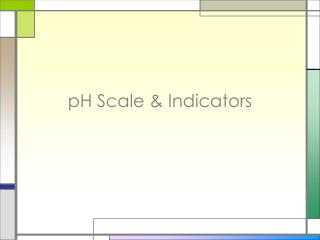# pH Scale & Indicators - PowerPoint PPT PresentationDownload PresentationpH Scale & Indicators

pH Scale & IndicatorsDownload Presentation## pH Scale & Indicators

- - - - - - - - - - - - - - - - - - - - - - - - - - - E N D - - - - - - - - - - - - - - - - - - - - - - - - - - -
##### Presentation Transcript

1. pH Scale & Indicators

2. pH Scale • pH Scale: • Expresses the strength of acids and bases • One pH unit = an inc/dec in [H3O+] by a ten fold • Neutral = 7 • Acid < 7 • Base > 7

3. Calculating pH pH = -log[H3O+] pOH = -log[OH-] pH + pOH = 14

4. Calculating the pH pH = - log [H+] (Remember that the [ ] mean Molarity) Example: If [H+] = 1 X 10-10pH = - log 1 X 10-10 pH = - (- 10) pH = 10 Example: If [H+] = 1.8 X 10-5pH = - log 1.8 X 10-5 pH = - (- 4.74) pH = 4.74

5. Calculating pH • What is the pH of 0.050 M HNO3? pH = -log[H3O+] pH = -log[0.050] pH = 1.3 Acidic or basic? Acidic

6. Calculating pH • What is the molarity of HBr in a solution that has a pOH of 9.6? pH + pOH = 14 pH + 9.6 = 14 pH = 4.4 pH = -log[H3O+] 4.4 = -log[H3O+] -4.4 = log[H3O+] [H3O+] = 4.0  10-5 M HBr Acidic

7. pH Indicators • Indicator: a compound that changes color in the presence of an acid or base • Most indicators are weak organic acids or bases that react with ions in solution.

8. pH Indicators • Two Common Indicators are • Litmus • Acids turn blue litmus red • Bases turn red litmus blue • Phenolphthalein • Colorless in acid • Faint pink in neutral • Bright pink in base pH Meter: a device used to measure the exact pH value of a solution

9. pH Scale – Color Chart

10. pH 4.0 pH 5.0 pH 7.0 pH 6.0 pH 8.0 pH 9.0 pH 10.0